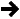IntroductionSpirit is an object-oriented recursive-descent parser generator framework implemented using template meta-programming techniques. Expression templates allow us to approximate the syntax of Extended Backus-Normal Form (EBNF) completely in C++.

The Spirit framework enables a target grammar to be written exclusively in C++. Inline EBNF grammar specifications can mix freely with other C++ code and, thanks to the generative power of C++ templates, are immediately executable. In retrospect, conventional compiler-compilers or parser-generators have to perform an additional translation step from the source EBNF code to C or C++ code.

A simple EBNF grammar snippet:

````    ````group       ::= '(' expression ')'
factor      ::= integer | group
term        ::= factor (('*' factor) | ('/' factor))*
expression  ::= term (('+' term) | ('-' term))*``````

is approximated using Spirit's facilities as seen in this code snippet:

````    ````group       = '(' >> expression >> ')';
factor      = integer | group;
term        = factor >> *(('*' >> factor) | ('/' >> factor));
expression  = term >> *(('+' >> term) | ('-' >> term));```
```

Through the magic of expression templates, this is perfectly valid and executable C++ code. The production rule expression is in fact an object that has a member function parse that does the work given a source code written in the grammar that we have just declared. Yes, it's a calculator. We shall simplify for now by skipping the type declarations and the definition of the rule integer invoked by factor. The production rule expression in our grammar specification, traditionally called the start symbol, can recognize inputs such as:

````    `12345
`    `-12345
`    `+12345
`    `1 + 2
`    `1 * 2
`    `1/2 + 3/4
`    `1 + 2 + 3 + 4
`    `1 * 2 * 3 * 4
`    `(1 + 2) * (3 + 4)
`    `(-1 + 2) * (3 + -4)
`    `1 + ((6 * 200) - 20) / 6
`    `(1 + (2 + (3 + (4 + 5))))
```

Certainly we have done some modifications to the original EBNF syntax. This is done to conform to C++ syntax rules. Most notably we see the abundance of shift >> operators. Since there are no 'empty' operators in C++, it is simply not possible to write something like:

````    `a b
```

as seen in math syntax, for example, to mean multiplication or, in our case, as seen in EBNF syntax to mean sequencing (b should follow a). The framework uses the shift >> operator instead for this purpose. We take the >> operator, with arrows pointing to the right, to mean "is followed by". Thus we write:

````    `a >> b
```

The alternative operator | and the parentheses () remain as is. The assignment operator = is used in place of EBNF's ::=. Last but not least, the Kleene star * which used to be a postfix operator in EBNF becomes a prefix. Instead of:

````    `a* //... in EBNF syntax,
```

we write:

````    `*a //... in Spirit.
```

since there are no postfix stars, "*", in C/C++. Finally, we terminate each rule with the ubiquitous semi-colon, ";".